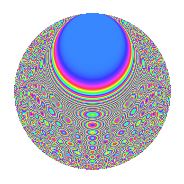# Properties

 Label 693.2.cgLevel 693 Weight 2 Character orbit cg Rep. character $$\chi_{693}(19,\cdot)$$ Character field $$\Q(\zeta_{30})$$ Dimension 304 Newform subspaces 3 Sturm bound 192 Trace bound 4

# Related objects

## Defining parameters

 Level: $$N$$ = $$693 = 3^{2} \cdot 7 \cdot 11$$ Weight: $$k$$ = $$2$$ Character orbit: $$[\chi]$$ = 693.cg (of order $$30$$ and degree $$8$$) Character conductor: $$\operatorname{cond}(\chi)$$ = $$77$$ Character field: $$\Q(\zeta_{30})$$ Newform subspaces: $$3$$ Sturm bound: $$192$$ Trace bound: $$4$$ Distinguishing $$T_p$$: $$2$$

## Dimensions

The following table gives the dimensions of various subspaces of $$M_{2}(693, [\chi])$$.

Total New Old
Modular forms 832 336 496
Cusp forms 704 304 400
Eisenstein series 128 32 96

## Trace form

 $$304q + 5q^{2} - 41q^{4} + 3q^{5} - 5q^{7} + 40q^{8} + O(q^{10})$$ $$304q + 5q^{2} - 41q^{4} + 3q^{5} - 5q^{7} + 40q^{8} + 3q^{11} - 4q^{14} + 13q^{16} + 45q^{17} - 15q^{19} + 52q^{22} + 14q^{23} - 31q^{25} - 51q^{26} + 20q^{28} - 27q^{31} + 75q^{35} + 7q^{37} - 9q^{38} - 105q^{40} + 52q^{44} - 20q^{46} + 21q^{47} + 23q^{49} - 30q^{50} - 15q^{52} - q^{53} + 112q^{56} - 6q^{58} - 45q^{59} - 30q^{61} - 132q^{64} - 36q^{67} - 105q^{68} + q^{70} + 12q^{71} - 60q^{73} - 85q^{74} - 30q^{79} - 153q^{80} + 63q^{82} - 190q^{85} - 84q^{86} - 21q^{88} - 6q^{89} - 20q^{91} + 174q^{92} - 15q^{94} - 40q^{95} + O(q^{100})$$

## Decomposition of $$S_{2}^{\mathrm{new}}(693, [\chi])$$ into newform subspaces

Label Dim. $$A$$ Field CM Traces $q$-expansion
$$a_2$$ $$a_3$$ $$a_5$$ $$a_7$$
693.2.cg.a $$48$$ $$5.534$$ None $$5$$ $$0$$ $$15$$ $$-5$$
693.2.cg.b $$128$$ $$5.534$$ None $$0$$ $$0$$ $$0$$ $$10$$
693.2.cg.c $$128$$ $$5.534$$ None $$0$$ $$0$$ $$-12$$ $$-10$$

## Decomposition of $$S_{2}^{\mathrm{old}}(693, [\chi])$$ into lower level spaces

$$S_{2}^{\mathrm{old}}(693, [\chi]) \cong$$ $$S_{2}^{\mathrm{new}}(77, [\chi])$$$$^{\oplus 3}$$$$\oplus$$$$S_{2}^{\mathrm{new}}(231, [\chi])$$$$^{\oplus 2}$$

## Hecke Characteristic Polynomials

There are no characteristic polynomials of Hecke operators in the database